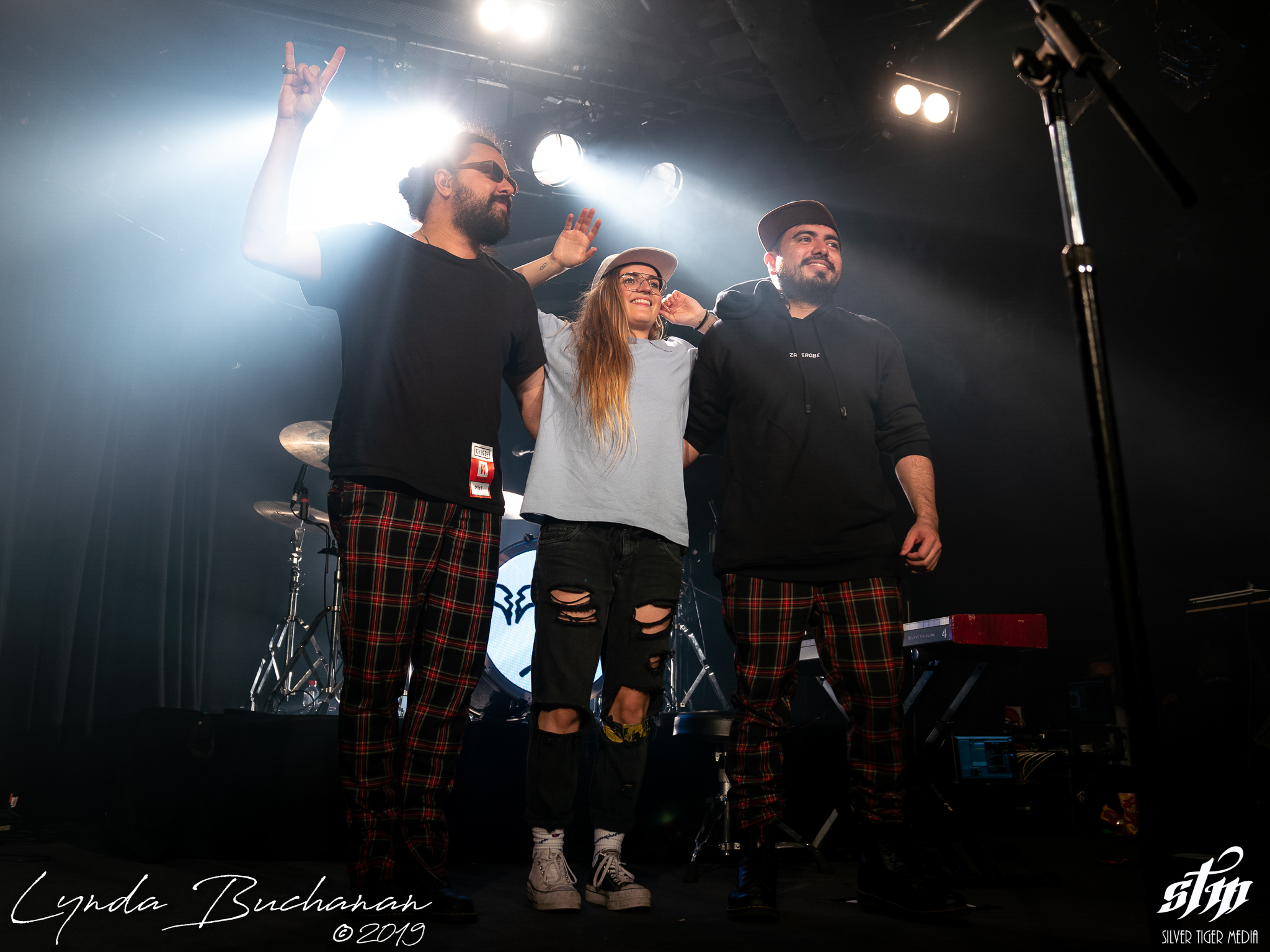# G Flip – Corner Hotel 9th May 2019

### G Flip – Corner Hotel 9th May 2019

G Flip played Melbourne’s Corner Hotel on 9th May 2019, shot for Silver Tiger Media.

G Flip (1 of 48)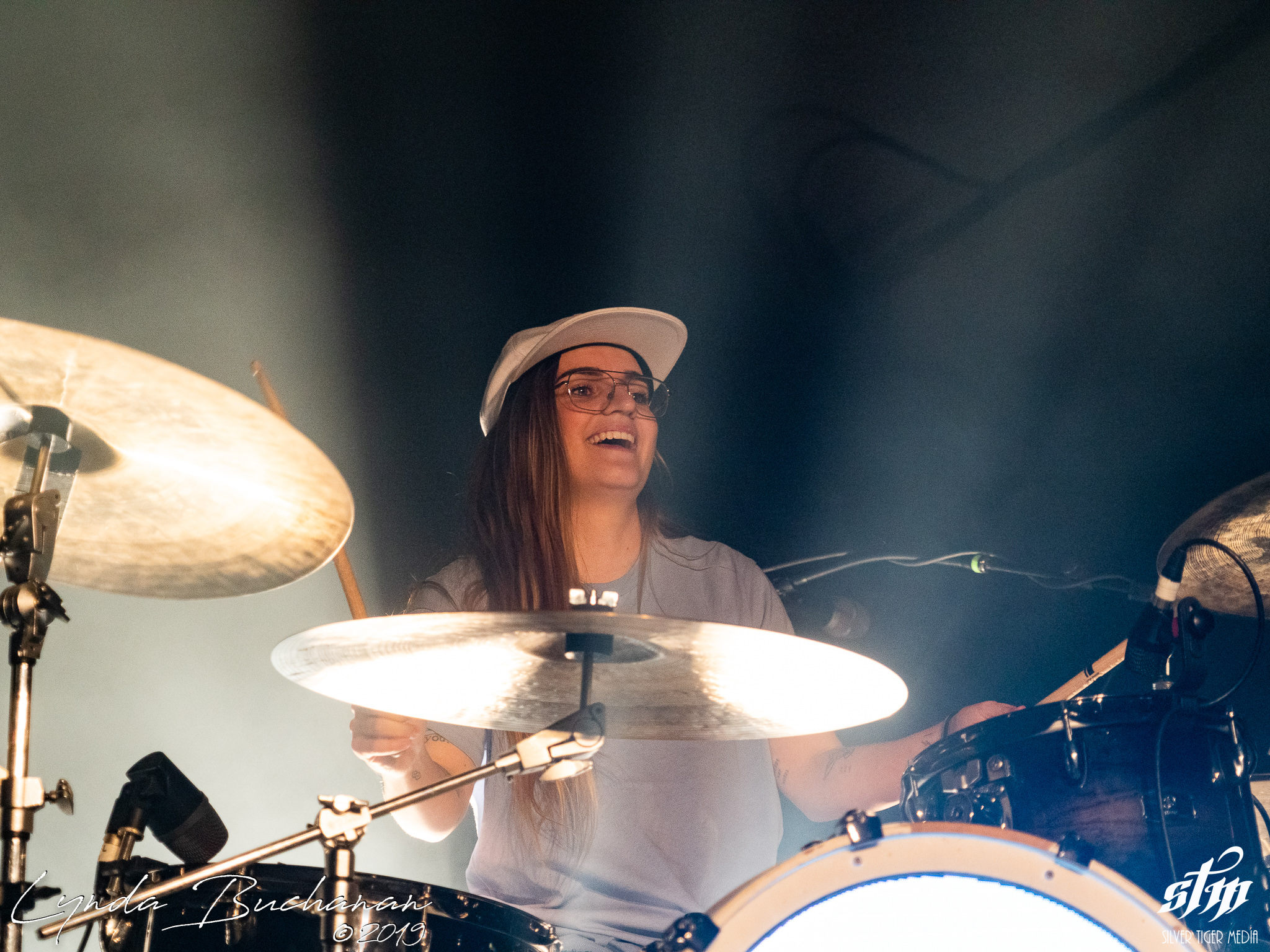G Flip (2 of 48)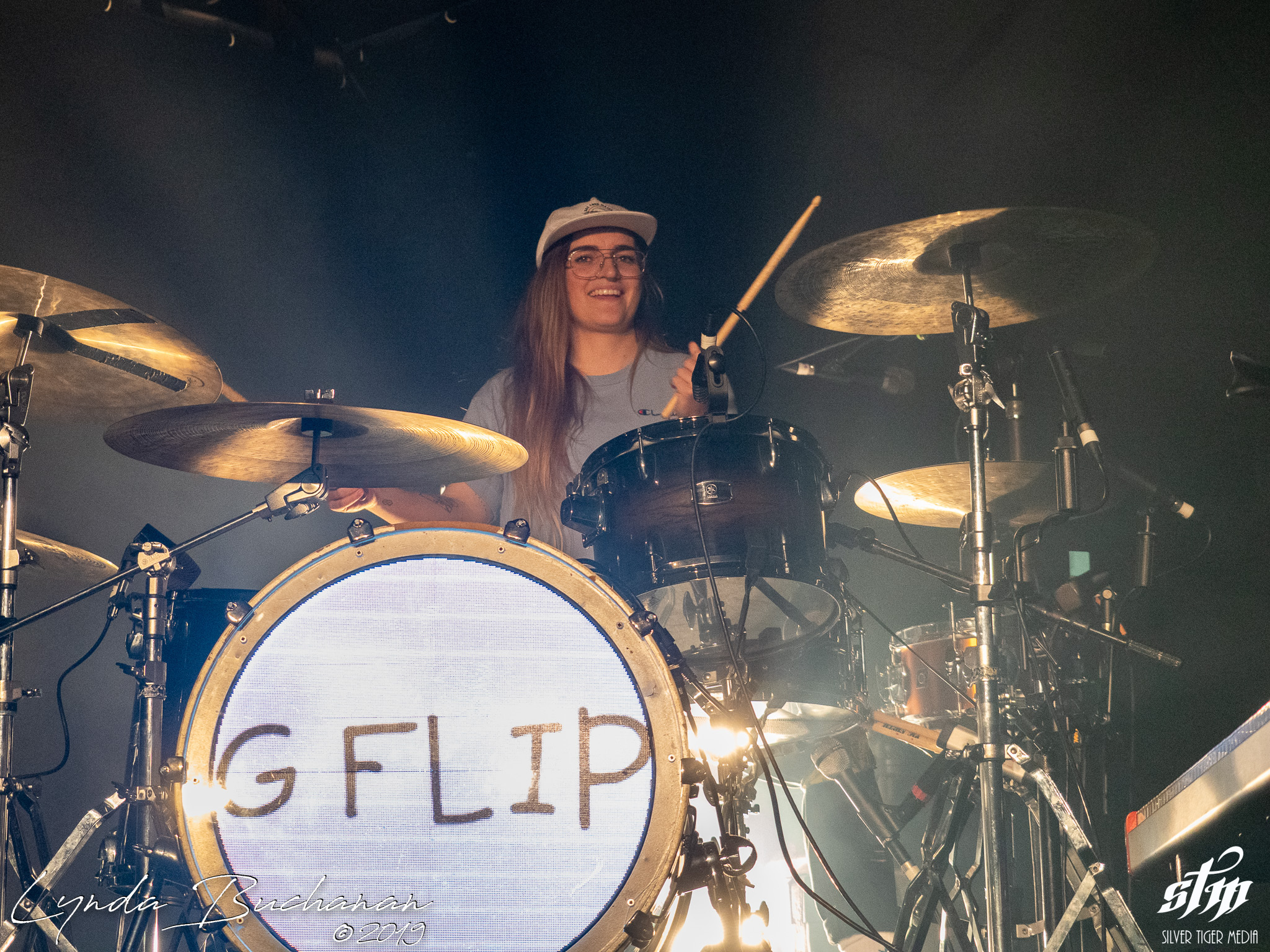G Flip (3 of 48)G Flip (4 of 48)G Flip (5 of 48)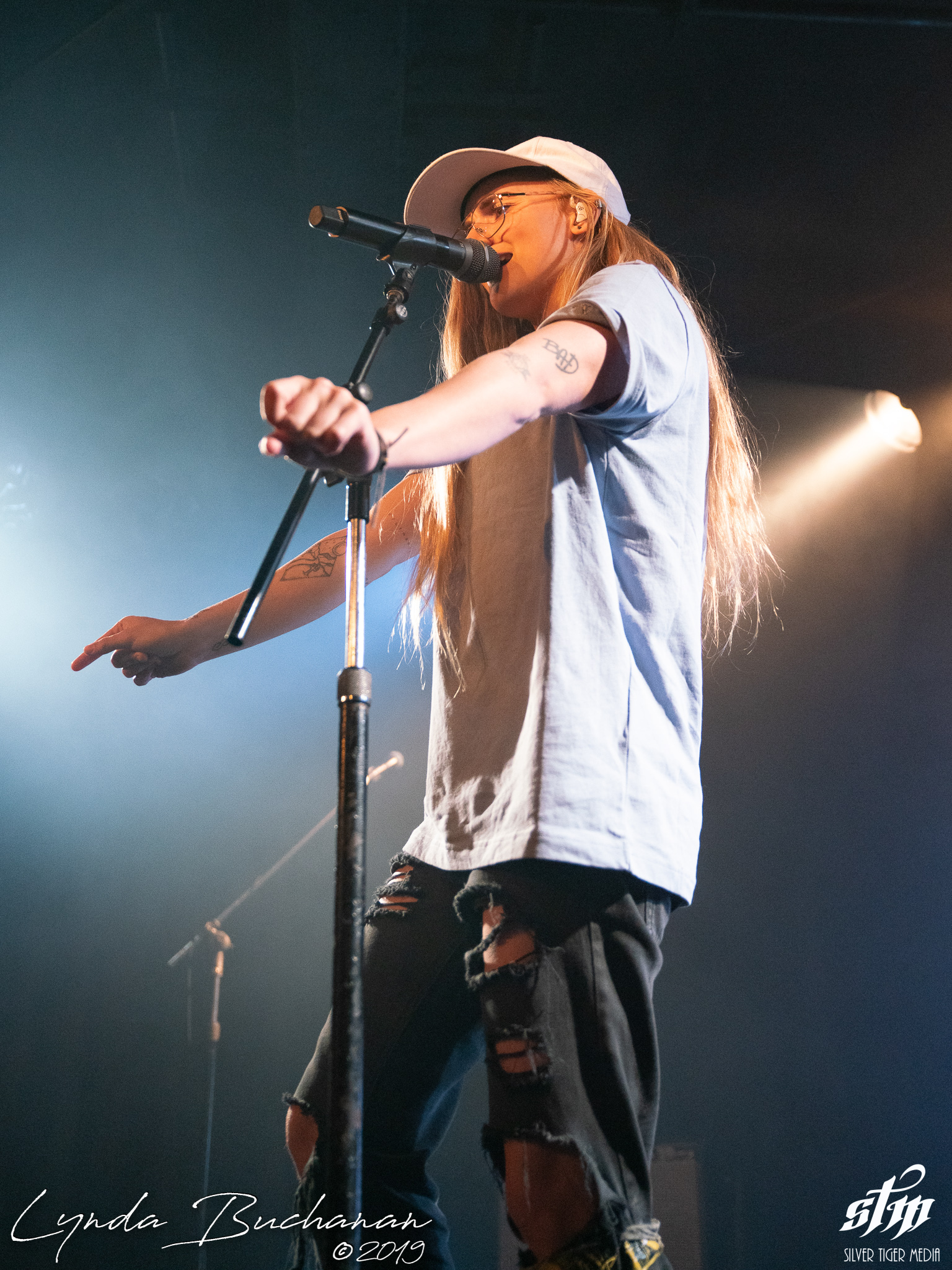G Flip (6 of 48)G Flip (7 of 48)G Flip (8 of 48)G Flip (9 of 48)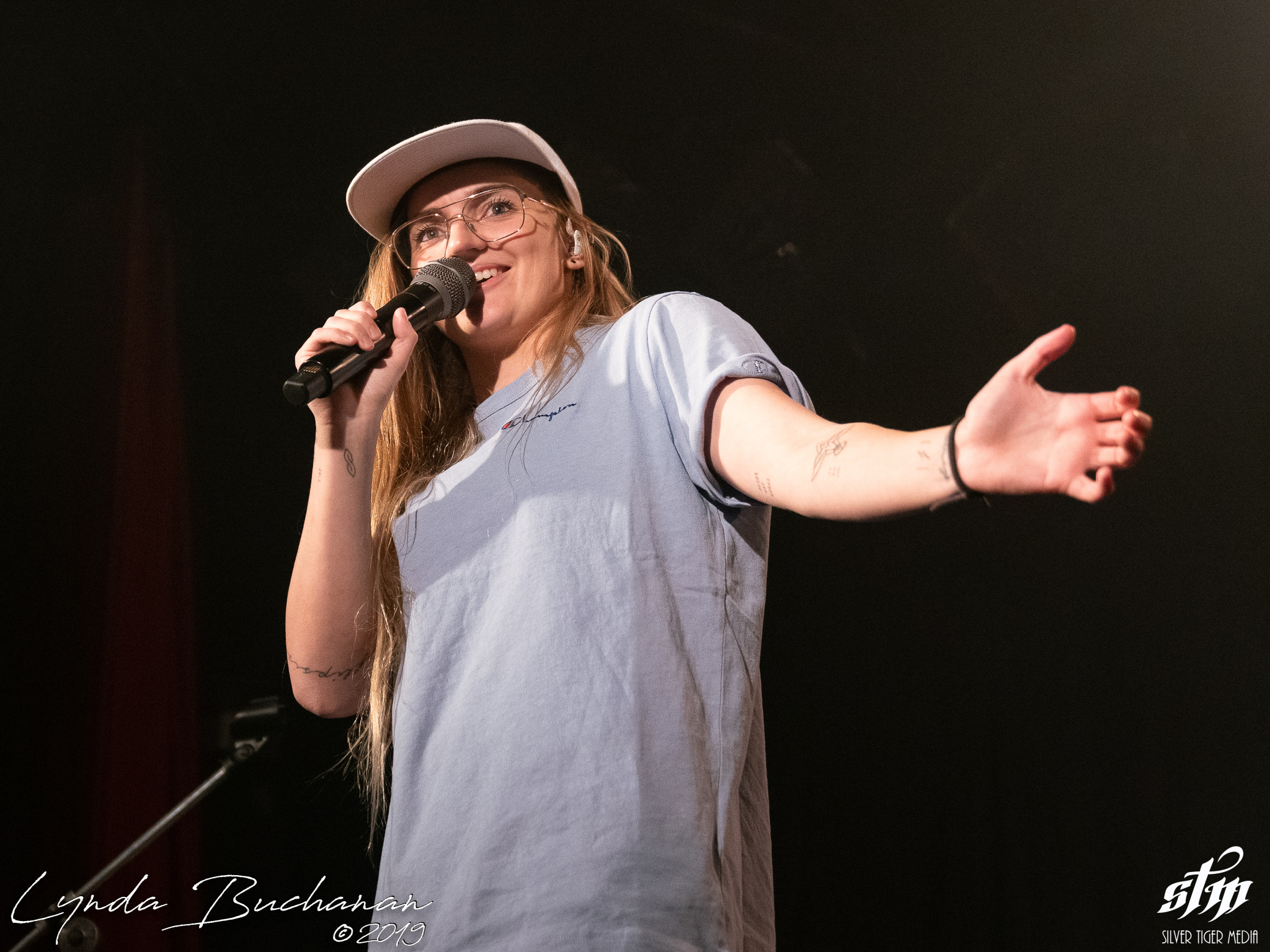G Flip (10 of 48)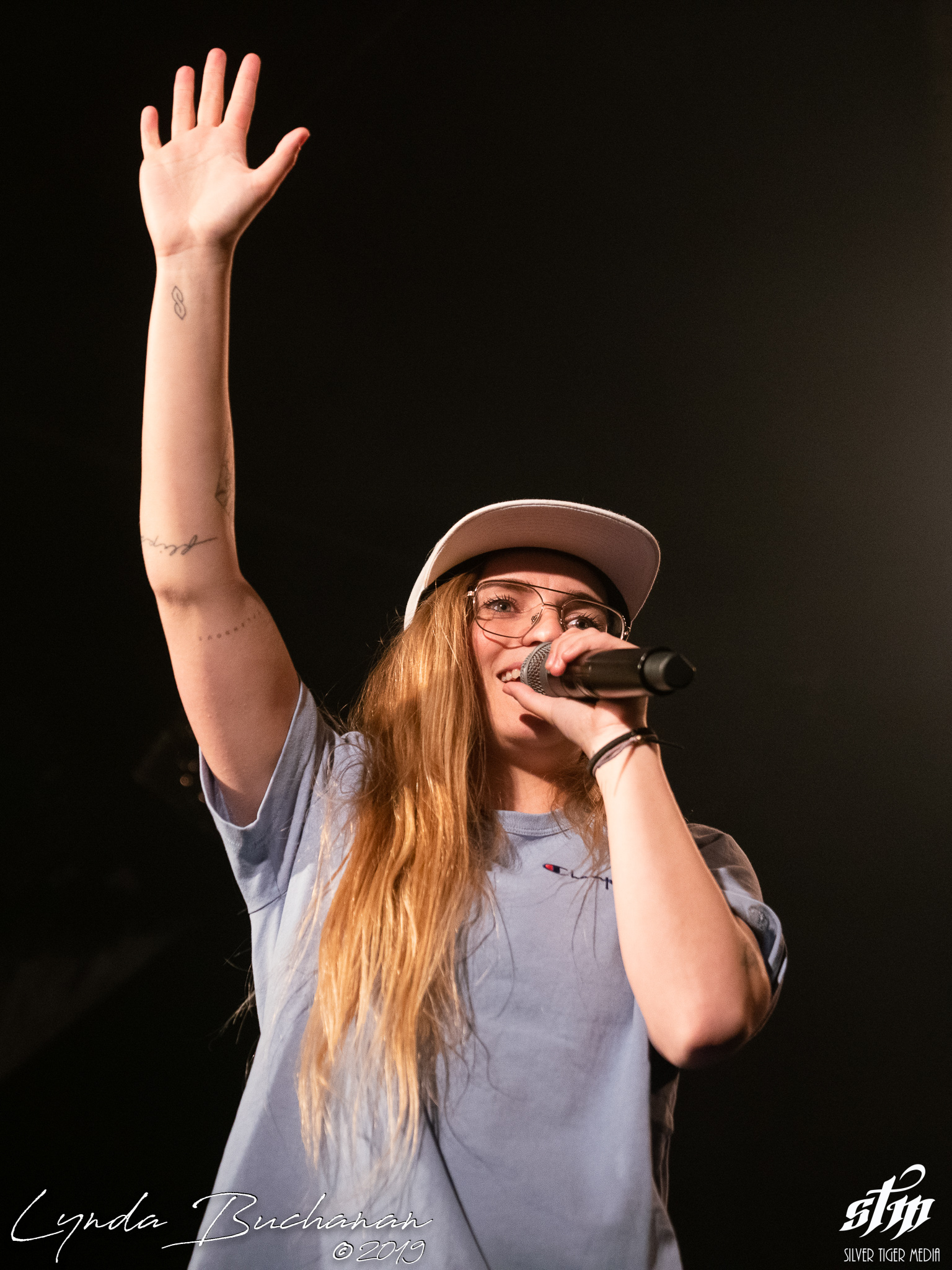G Flip (11 of 48)G Flip (12 of 48)G Flip (13 of 48)G Flip (14 of 48)G Flip (15 of 48)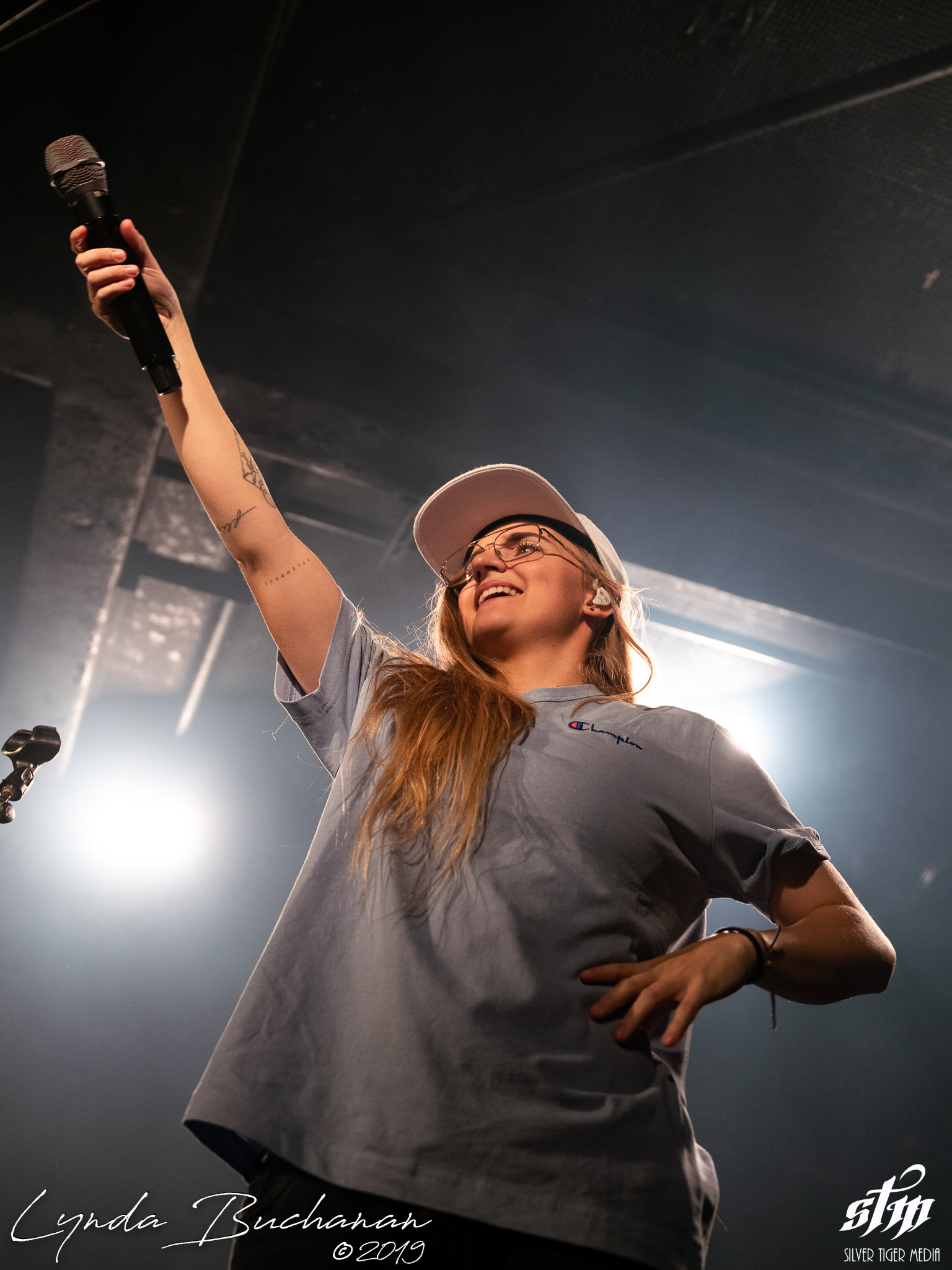G Flip (16 of 48)G Flip (17 of 48)G Flip (18 of 48)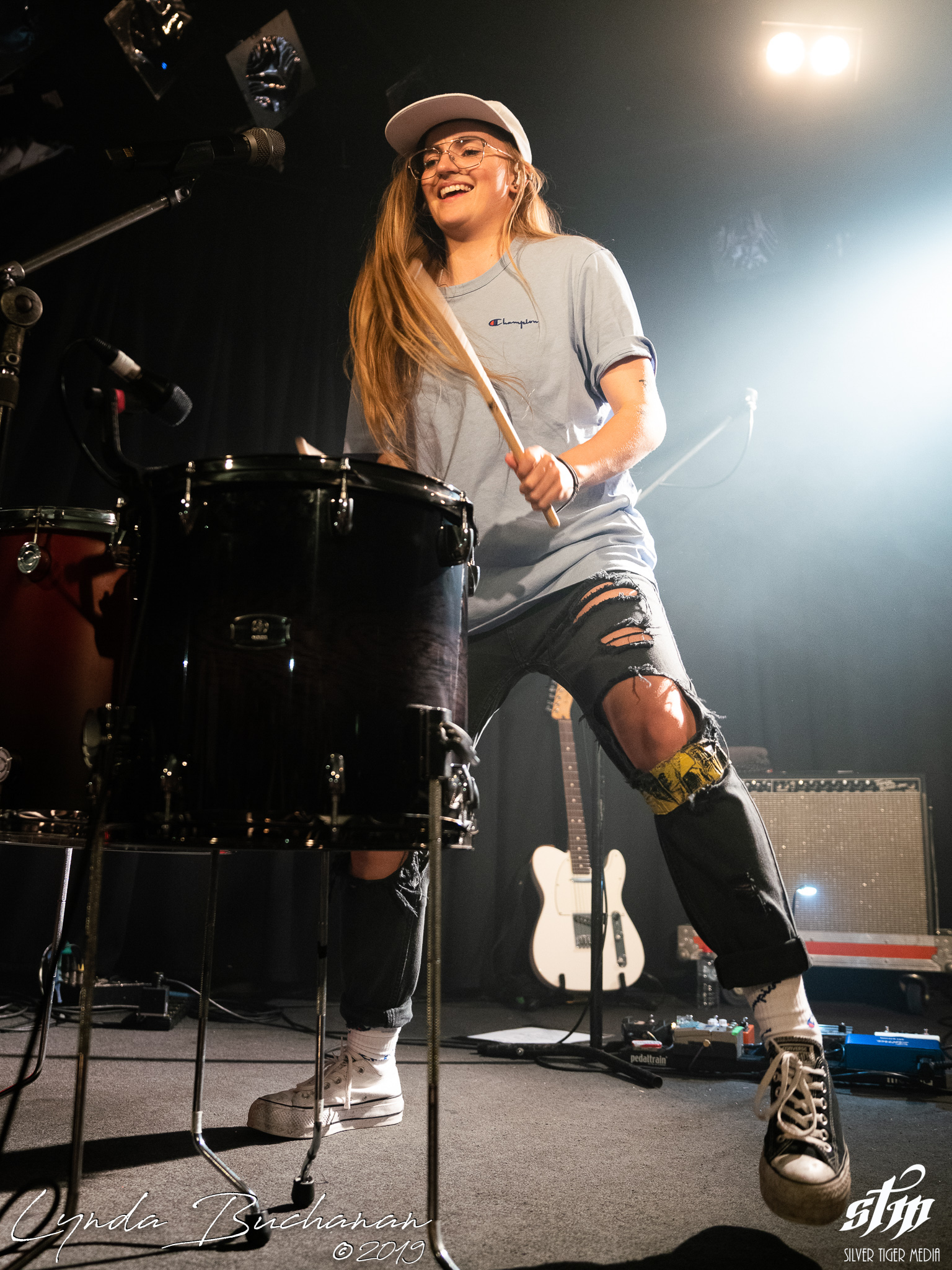G Flip (19 of 48)G Flip (20 of 48)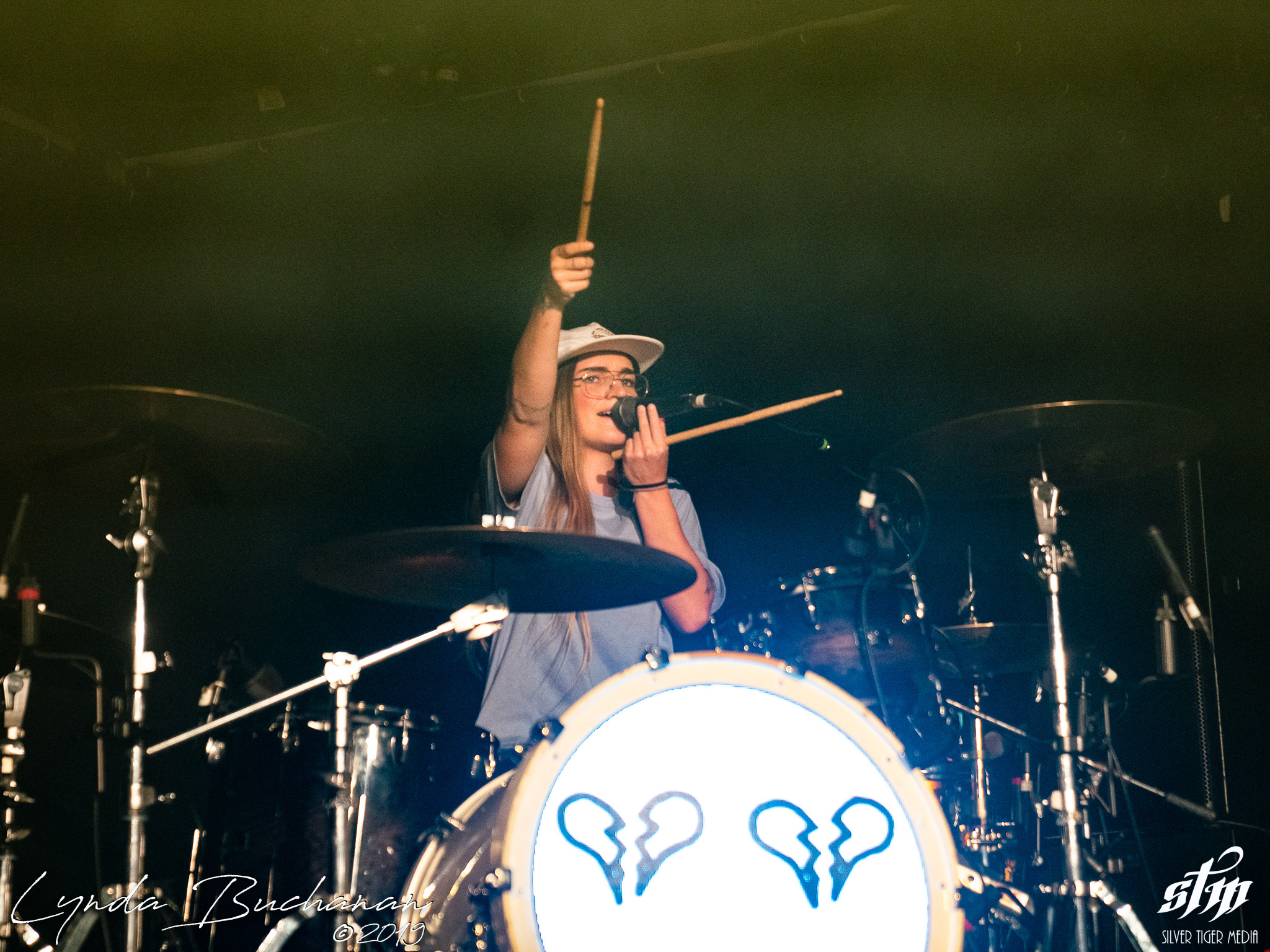G Flip (21 of 48)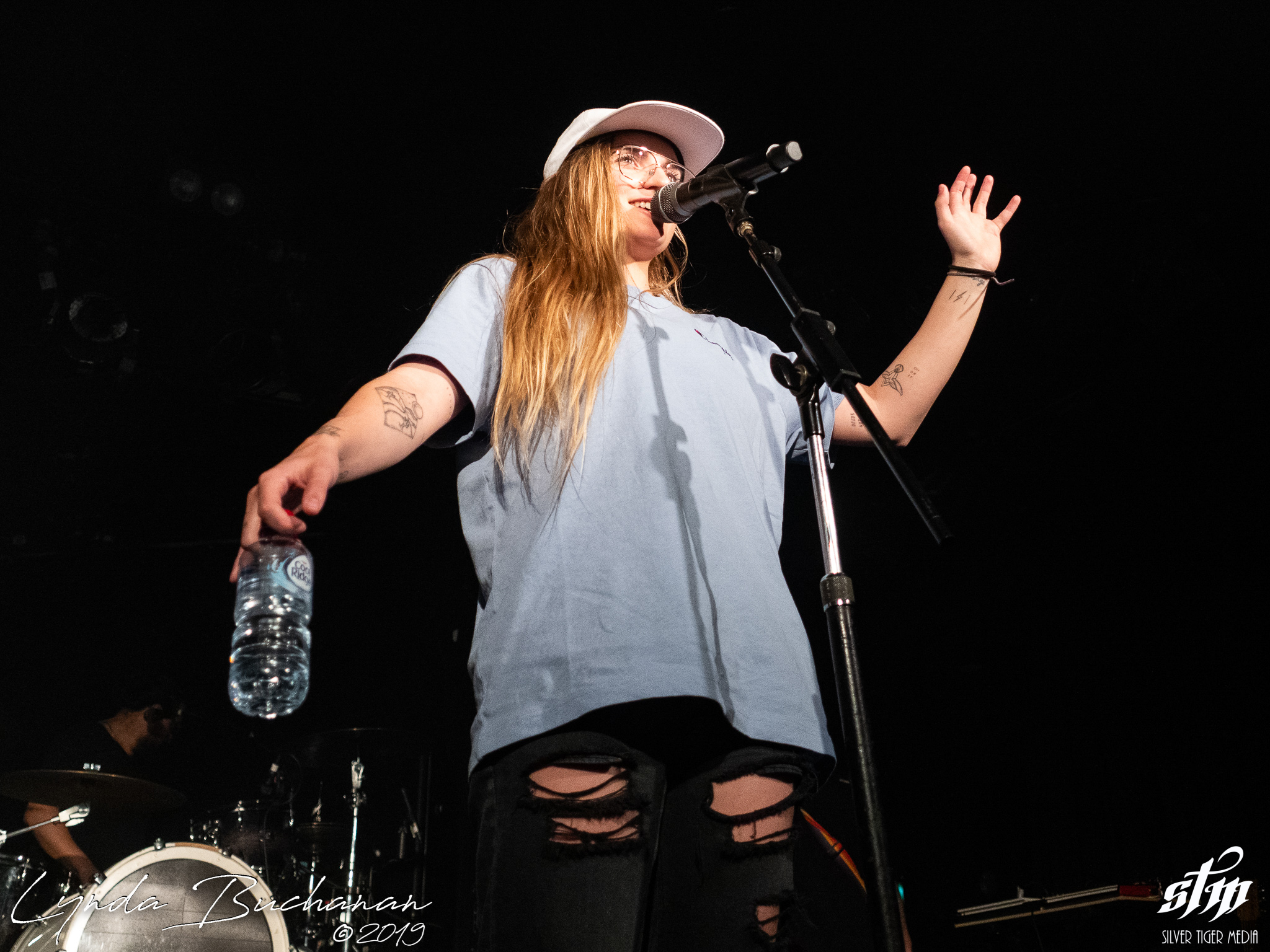G Flip (22 of 48)G Flip (23 of 48)G Flip (24 of 48)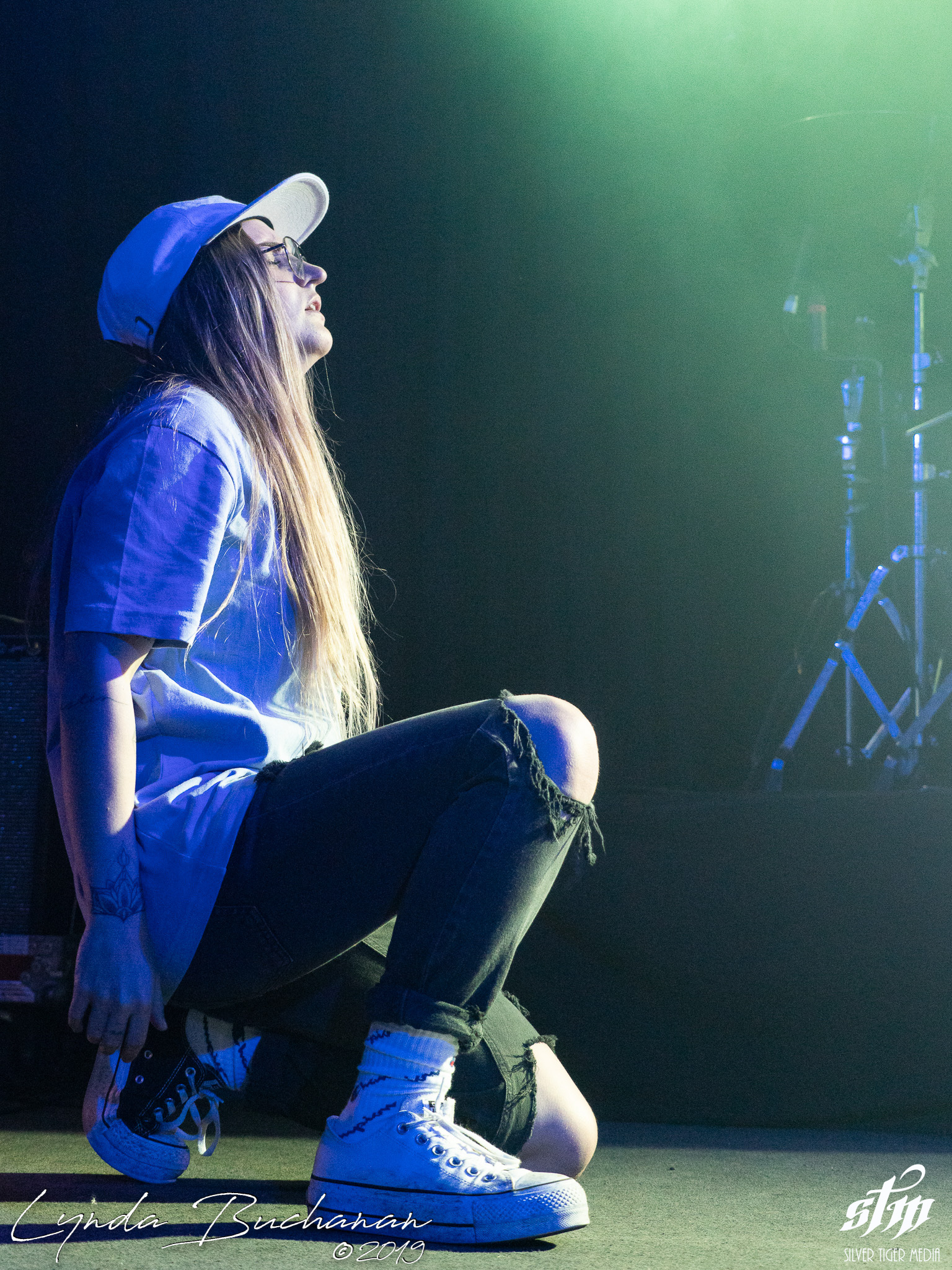G Flip (25 of 48)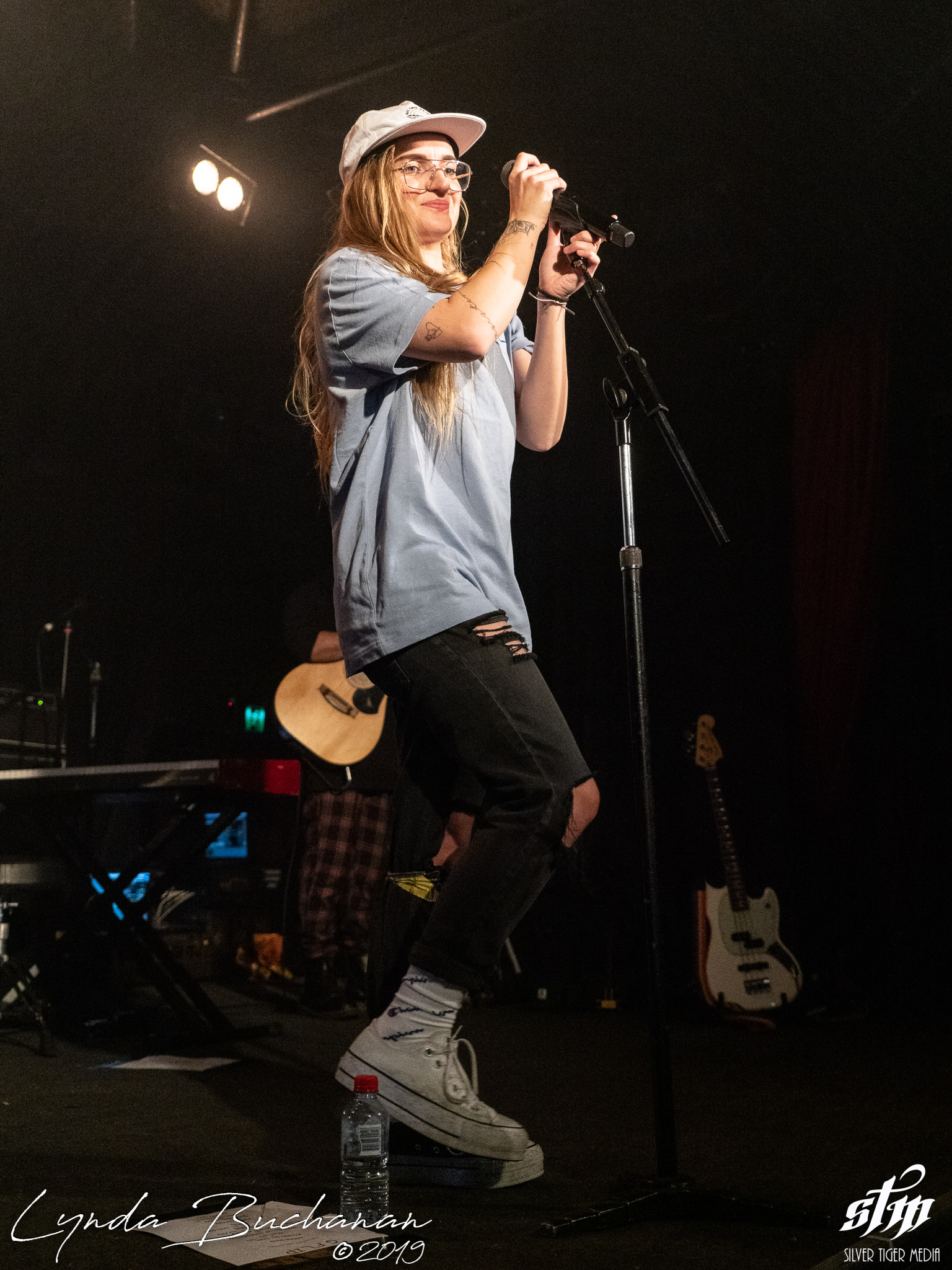G Flip (26 of 48)G Flip (27 of 48)G Flip (28 of 48)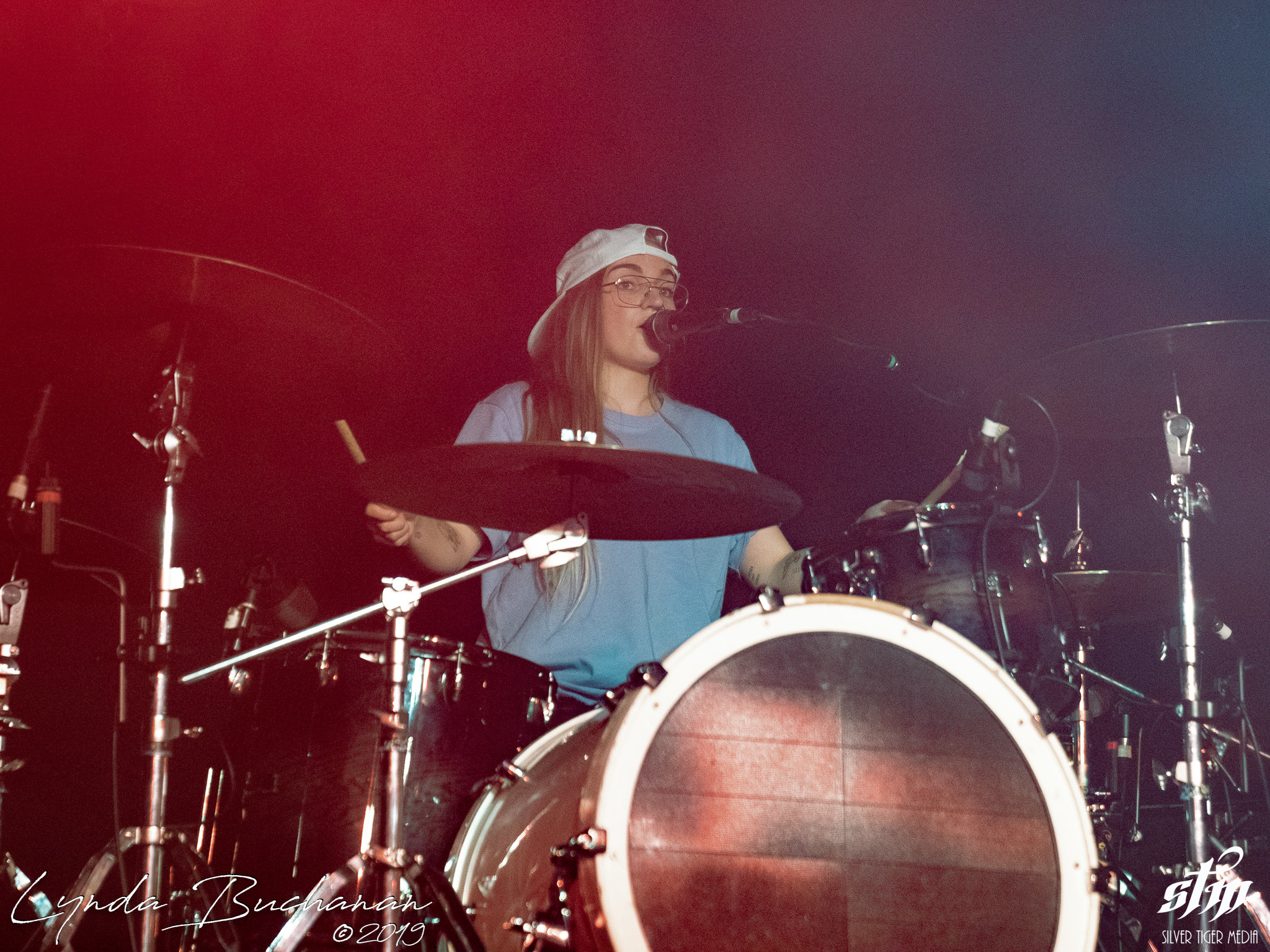G Flip (29 of 48)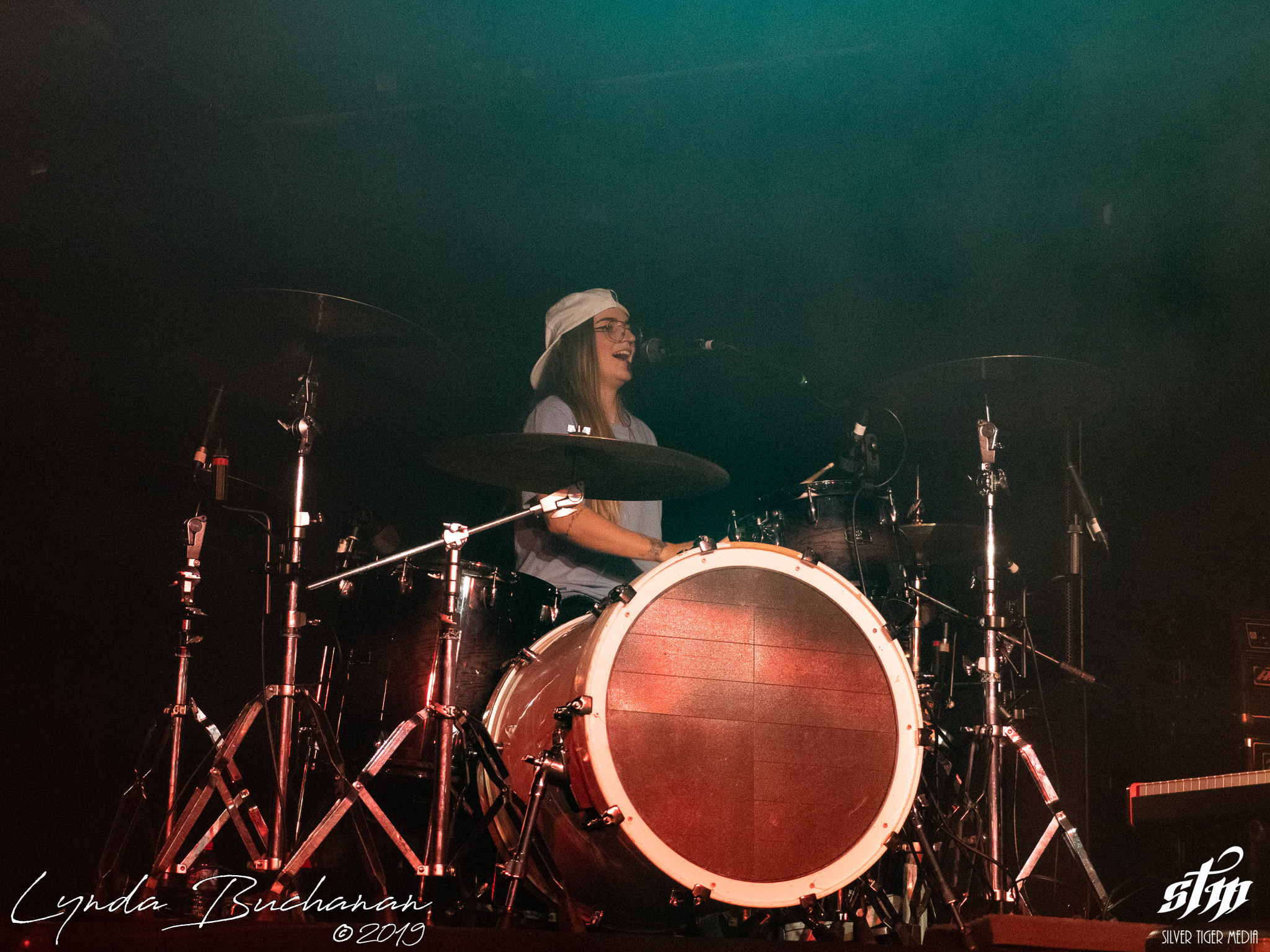G Flip (30 of 48)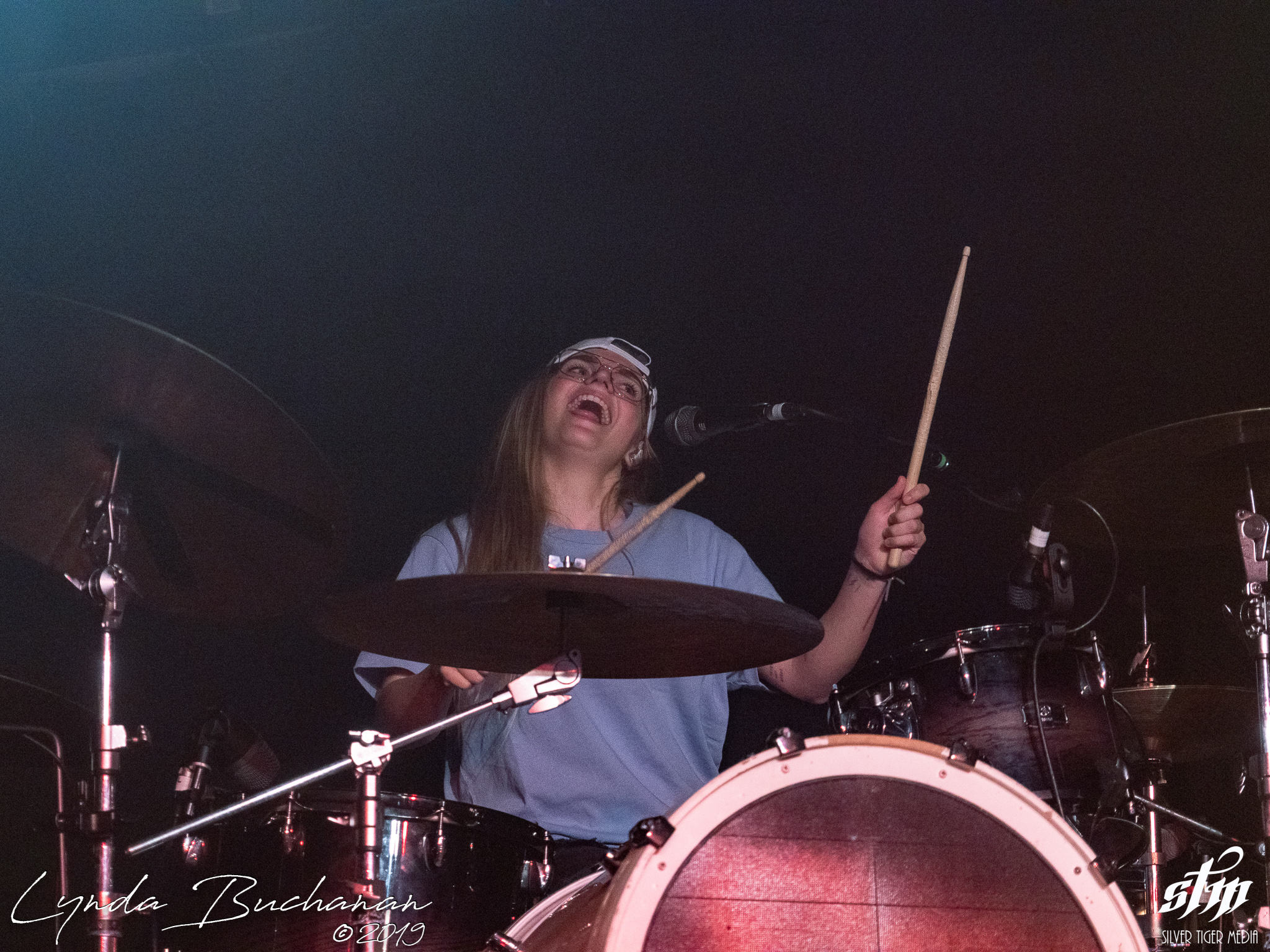G Flip (31 of 48)G Flip (32 of 48)G Flip (33 of 48)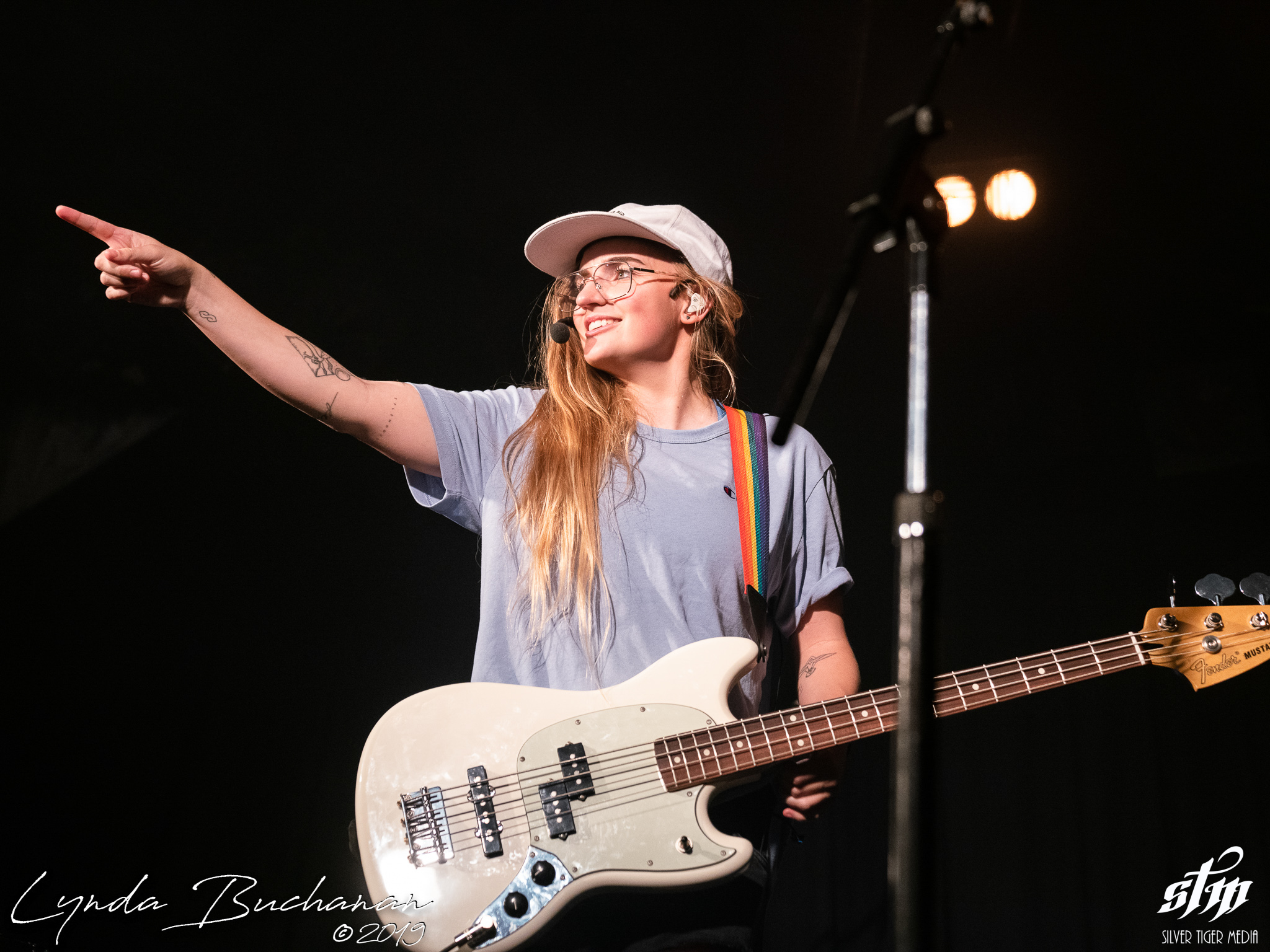G Flip (34 of 48)G Flip (35 of 48)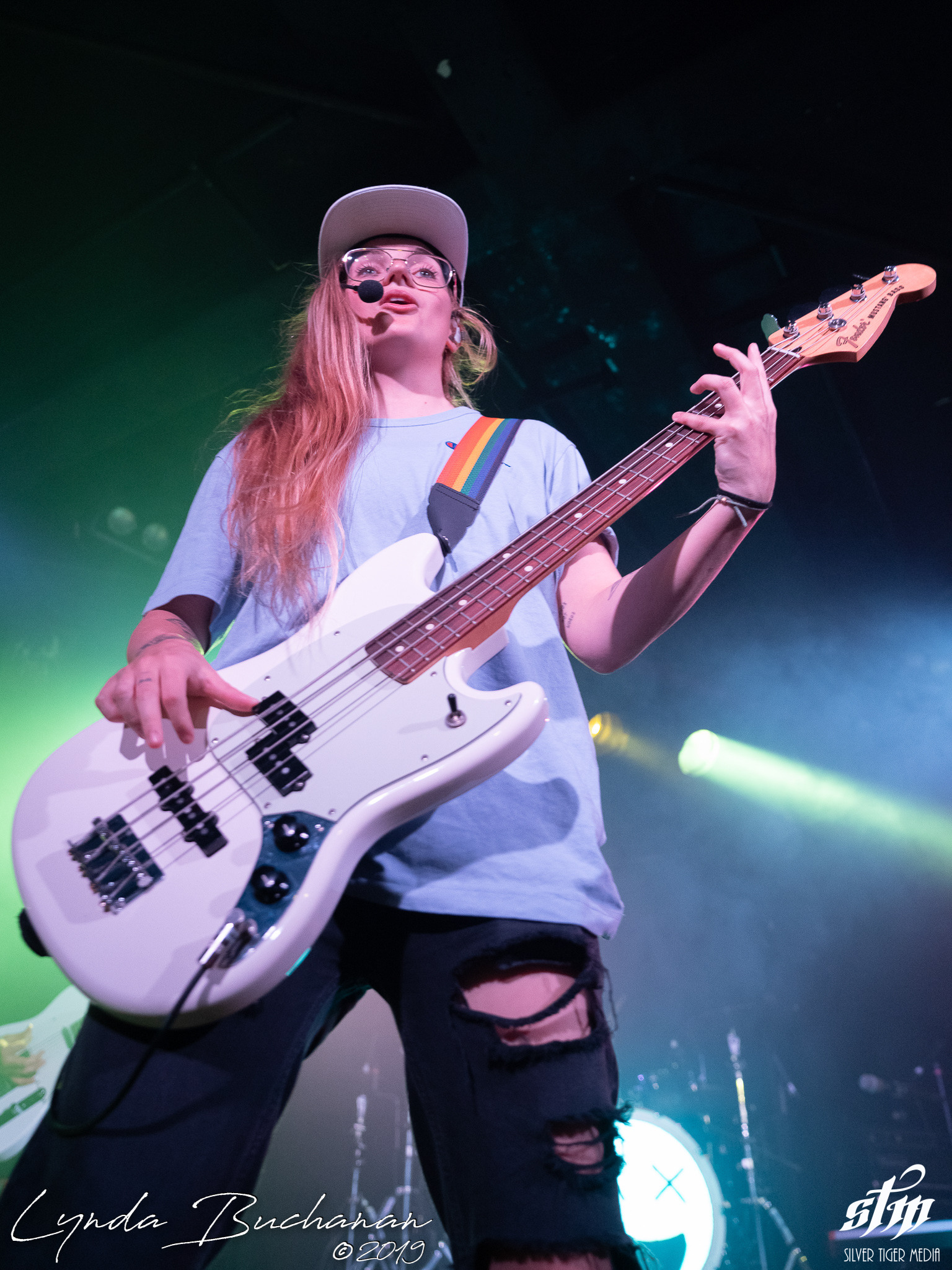G Flip (36 of 48)G Flip (37 of 48)G Flip (38 of 48)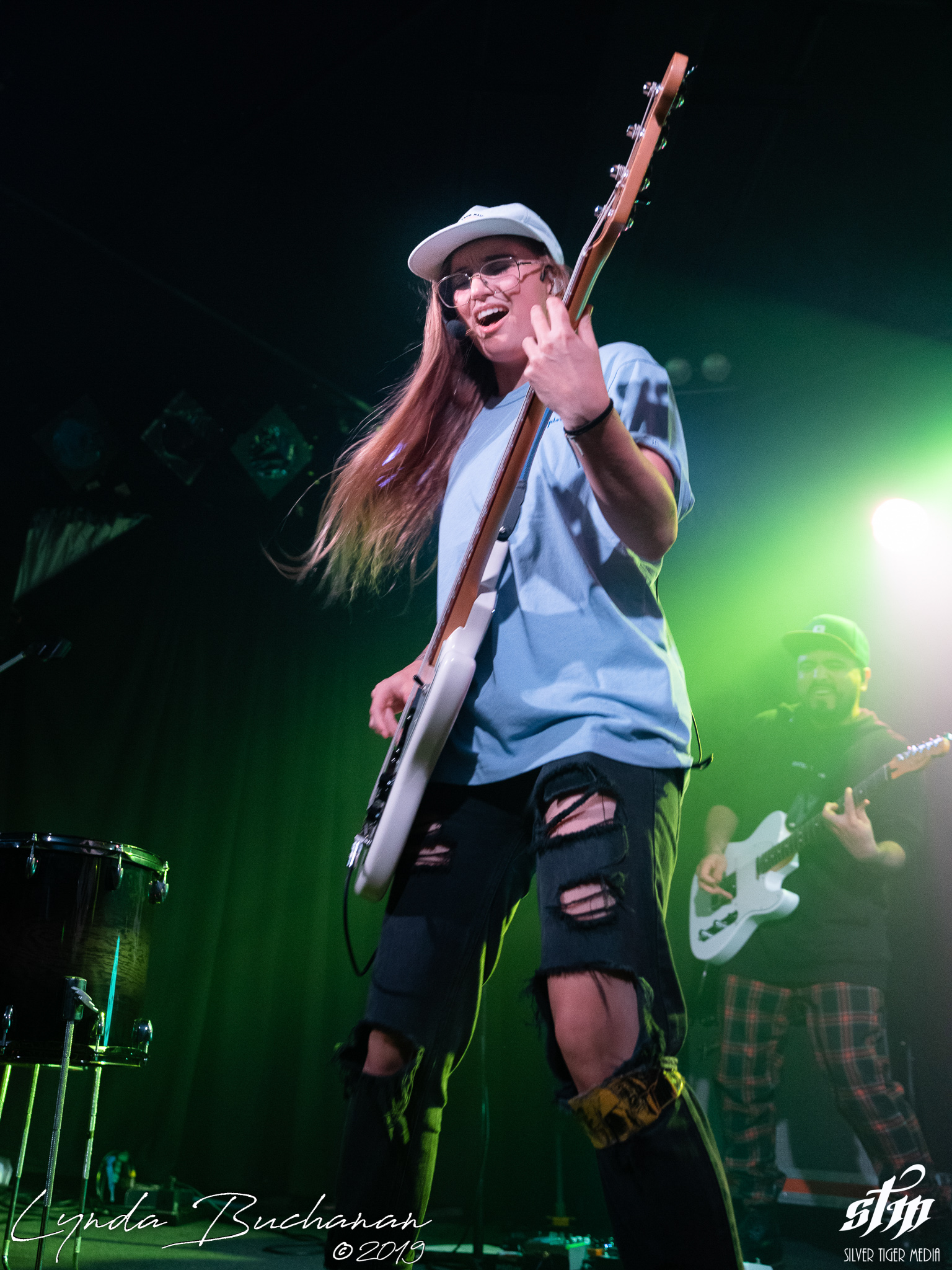G Flip (39 of 48)G Flip (40 of 48)G Flip (41 of 48)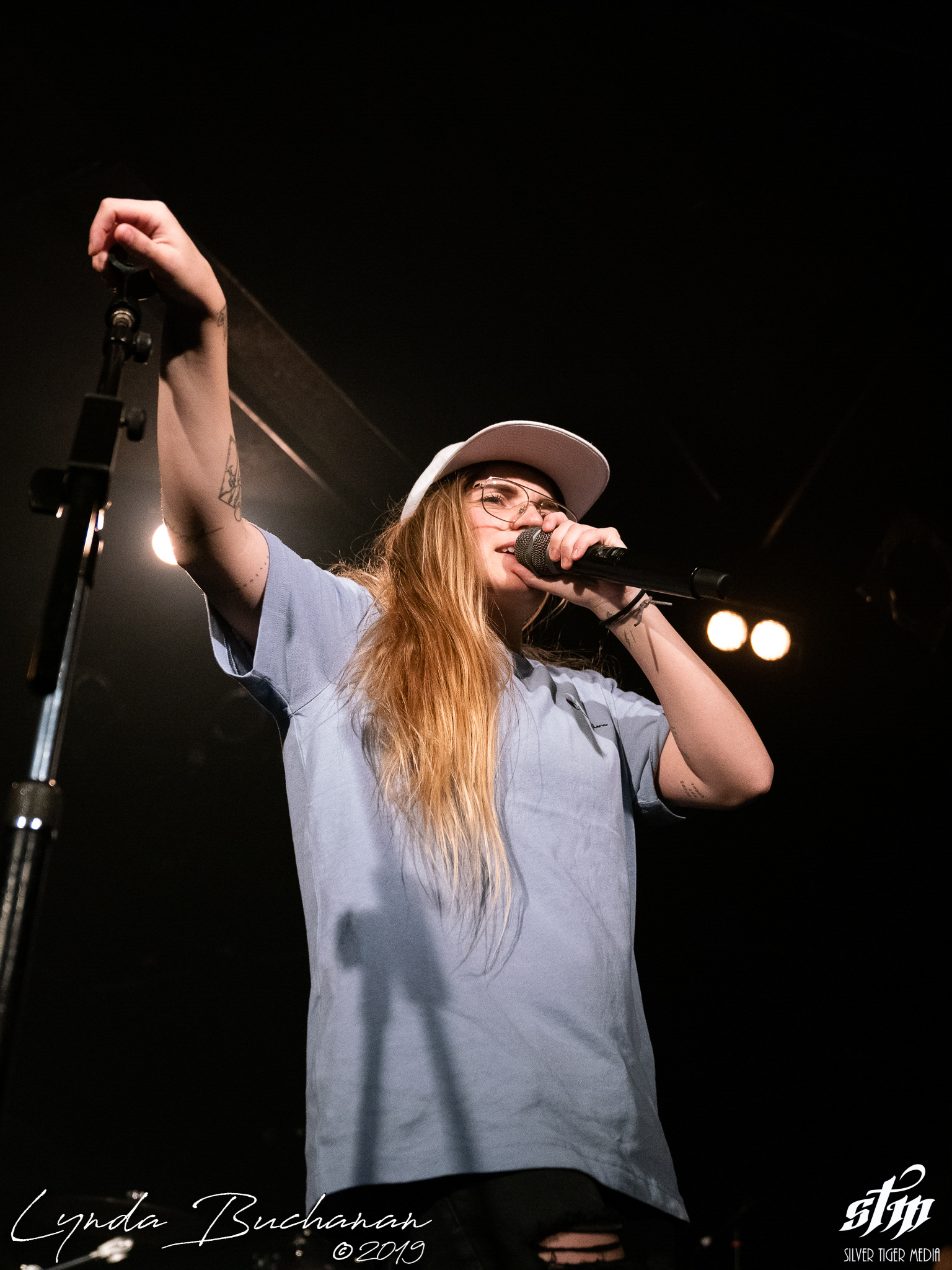G Flip (42 of 48)G Flip (43 of 48)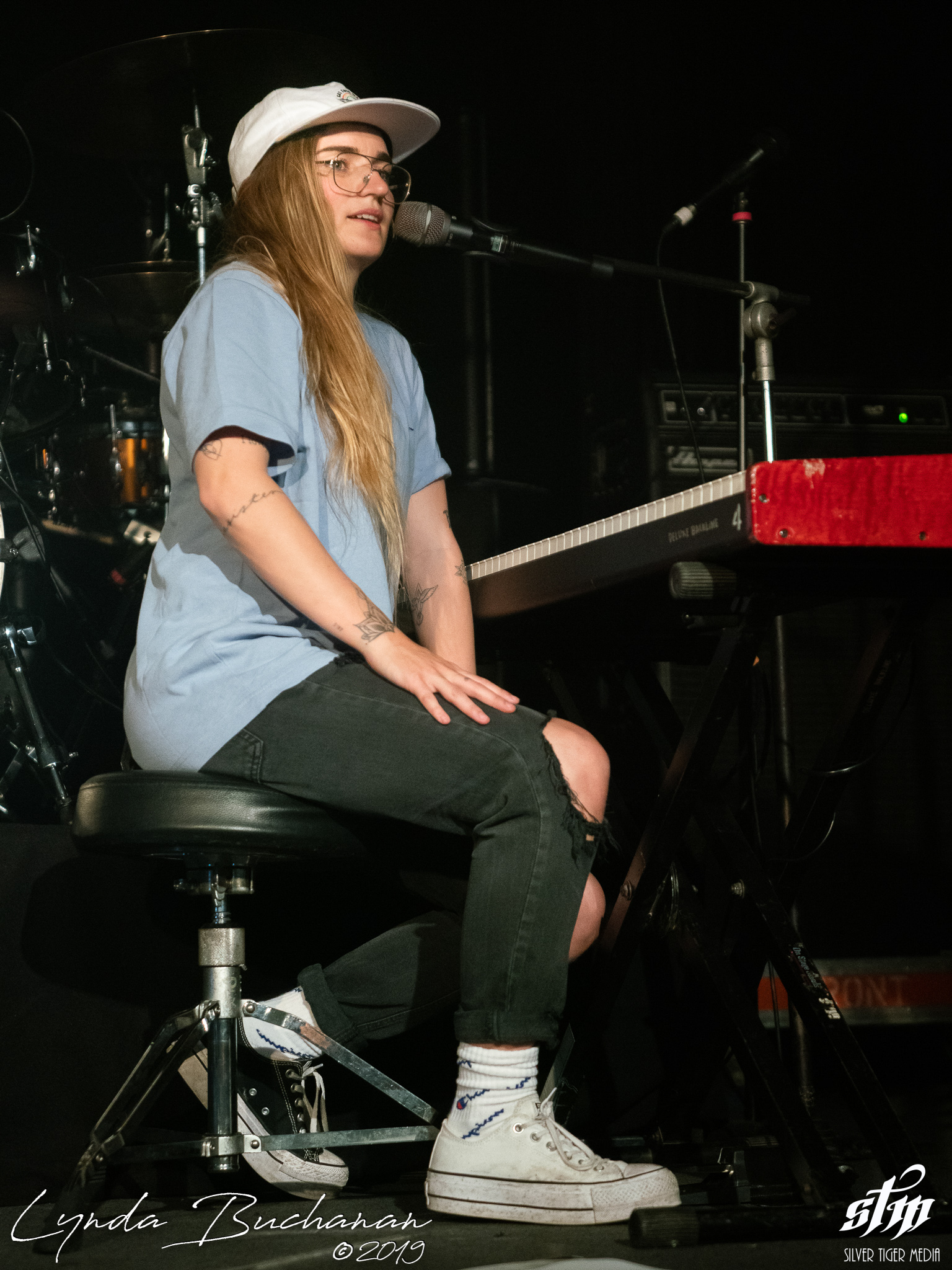G Flip (44 of 48)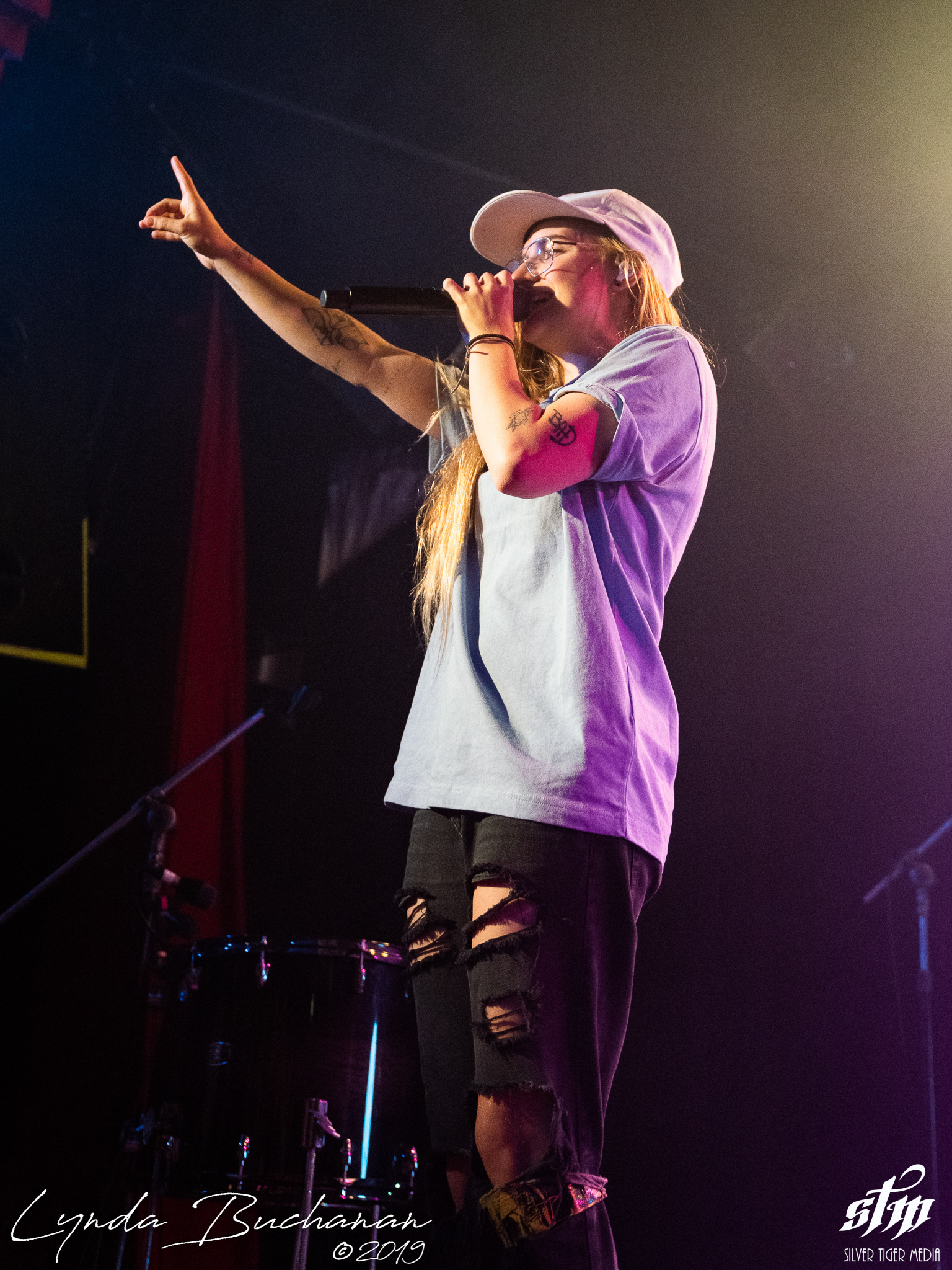G Flip (45 of 48)G Flip (46 of 48)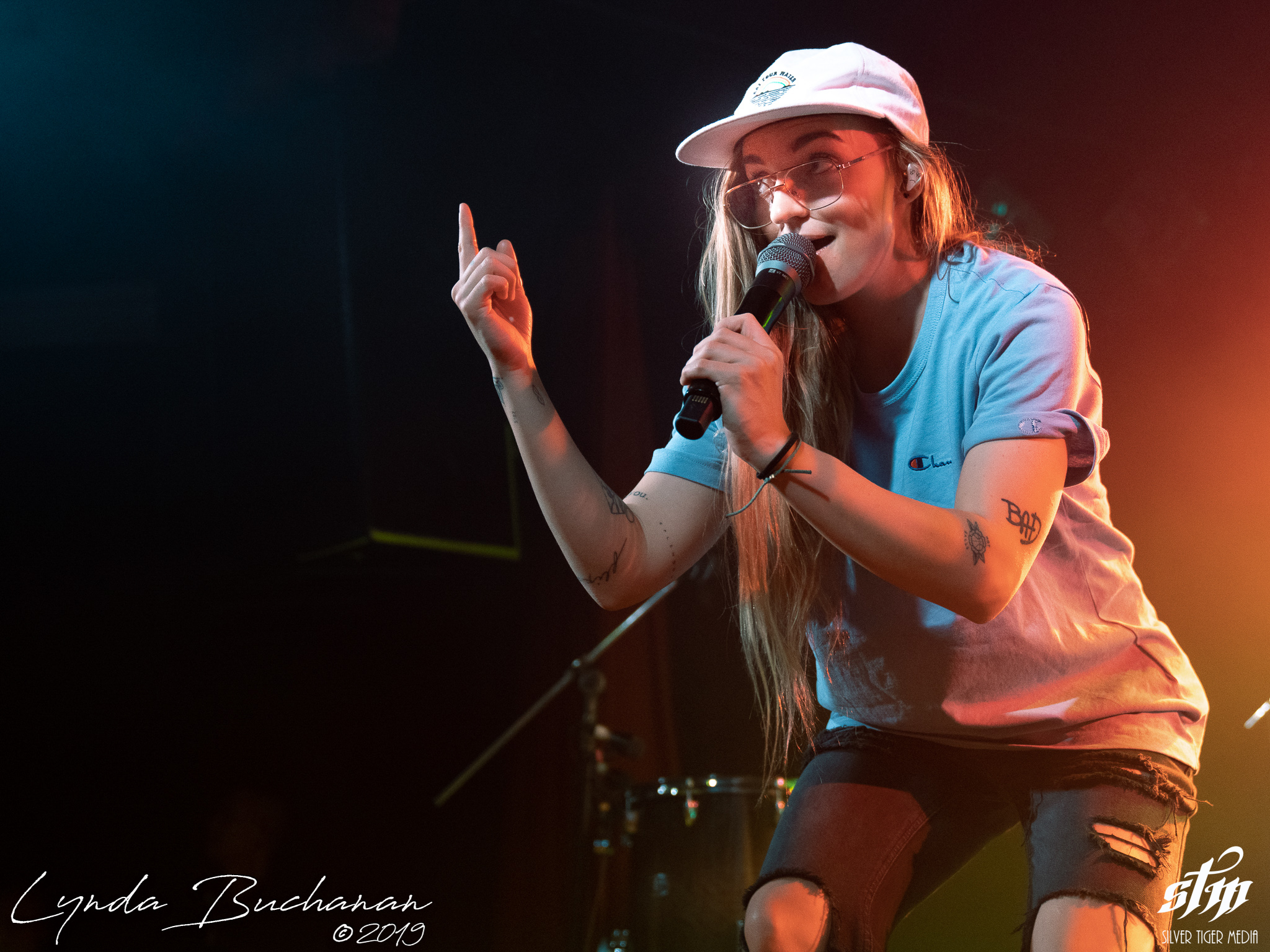G Flip (47 of 48)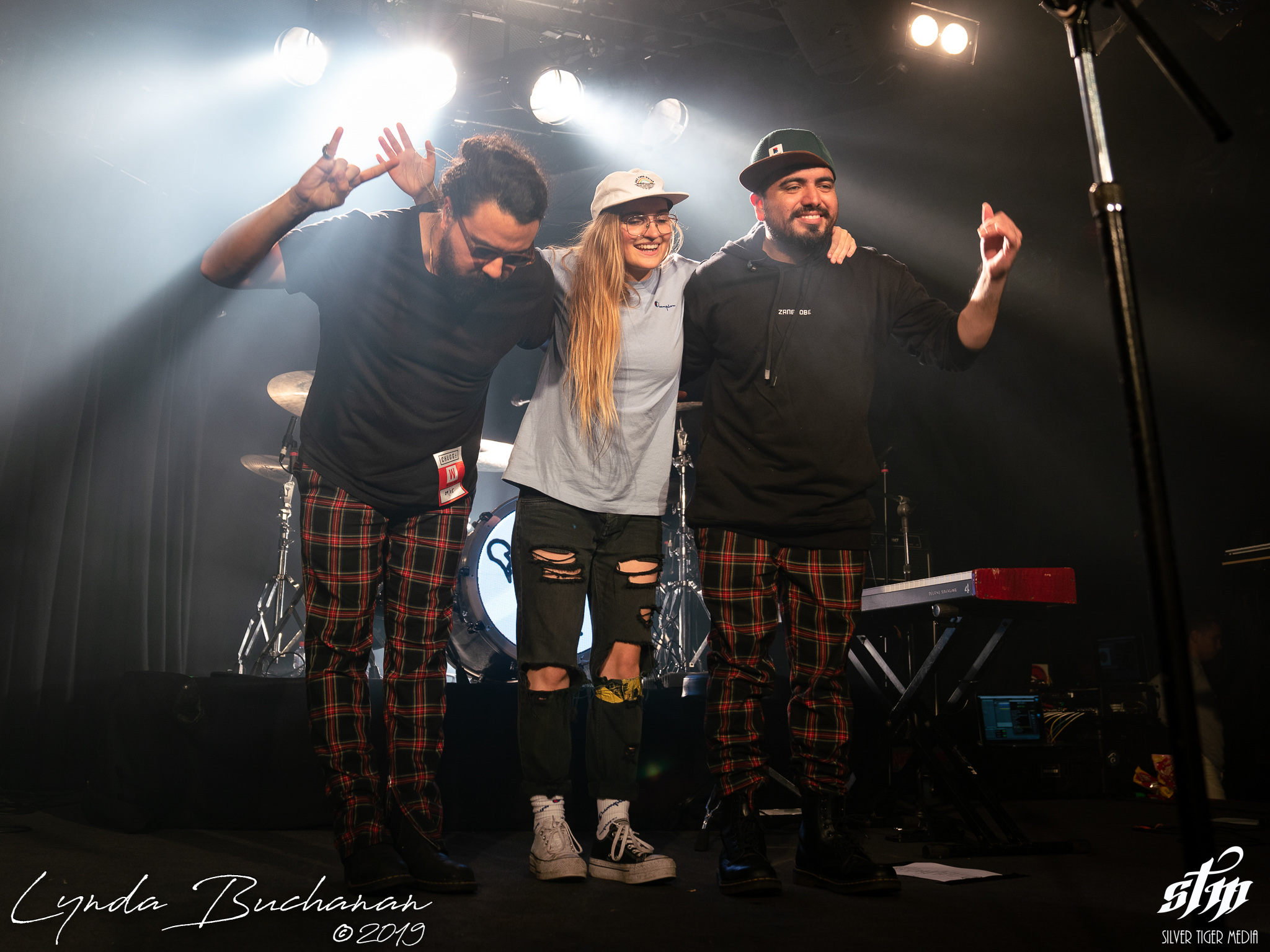G Flip (48 of 48)